# Electronics and Communication Engineering - Microwave Communication

### Exercise :: Microwave Communication - Section 4

26.

When resonant cavities are coupled together the result is

 A. one resonant frequency B. n resonant frequencies C.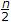resonant frequencies D. either (b) or (c)

Explanation:

No answer description available for this question. Let us discuss.

27.

For a rectangular waveguide having width a and height b, the cutoff wavelength for TM11 mode is equal to

 A.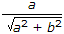B.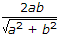C.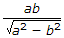D.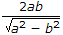Explanation:

No answer description available for this question. Let us discuss.

28.

The path length between ports 1 and 2 for the two waves in a hybrid ring is

 A. 1 B.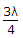C.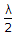D.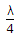Explanation:

The quantities required to be measured in microwave circuits are frequency, power and impedance.

Microwave frequency measurement is done by slotted line, resonant cavities and transfer oscillator.

Microwave power measurement uses bolometers and microwave power meters.

A self balancing bridge working on the principle of power substitution is commonly used.

Microwave impedance measurement is done by measurement of reflection coefficient and VSWR.

A slotted line and probe is a basic tool for these measurements.' Network analyser enables rapid impedance measurement over a broad frequency range.

29.

The radiation resistance of a circular loop of one turn is 0.01 ohm. For 5 turn loop the radiation resistance is

 A. 0.002 Ω B. 0.01 Ω C. 0.05 Ω D. 0.25 Ω

Explanation:

No answer description available for this question. Let us discuss.

30.

As wavelength decrease the size of high directivity antenna

 A. decreases B. increases C. is not affected D. either (b) or (c)